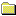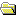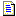Products Download Events Support Videos All Product Families ARM7, ARM9, and Cortex-M3 Products C16x, XC16x, and ST10 Products C251 and 80C251 Products Cx51 and 8051 Products Modified Anytime In the Last 7 Days In the Last 14 Days In the Last 30 Days In the Last 90 Days In the Last 6 Months

Technical Support

On-Line Manuals

C166 User's GuideC166 IntroductionCompiling ProgramsLanguage ExtensionsPreprocessorAdvanced ProgrammingSections, Classes, and GroupsSpecial SectionsLocating SectionsCustomization FilesStartup CodeSTARTUP.A66START167.A66START_V2.A66START_V3.A66Basic I/OMemory AllocationTRAPS.COptimizerOptimizer OptionsGeneral Optimizations80C166-Specific OptimizationsGlobal Register ColoringDPP RegistersStacksSystem StackUser StackStack SizesSystem Stack SizeUser Stack SizeXBUS Memory and PeripheralsOn-chip XRAMXBUS PeripheralsInterfacing C to AssemblerFunction ParametersFunction Return ValuesUsing the SRC DirectiveRegister UsageExample RoutinesTINY ModelSMALL ModelCOMPACT ModelMEDIUM ModelLARGE ModelData Storage FormatsBit Variables1-byte Scalars2-byte Scalars4-byte Scalarsfloat Scalarsfloat Errorsdouble Scalarsdouble Errorsnear Pointersfar Pointershuge Pointersxhuge PointersAbsolute Memory AccessAbsolute Access MacrosLinker Location ControlsDebuggingError MessagesLibrary ReferenceAppendix

# <!-- var kmNextPage = "c166_ap_doubleerror.htm"; var kmPrevPage = "c166_ap_floaterror.htm"; var kmNextPageTitle = "double Errors"; var kmPrevPageTitle = "float Errors"; var kmBreadCrumbs = "<a href=\"default.htm\">Home</a> &raquo; <a href=\"c166_advanced.htm\">Advanced Programming</a> &raquo; <a href=\"c166_ap_datastorage.htm\">Data Storage Formats</a> &raquo; double Scalars"; kmSetupPaging (); kmNavButtons(); //--> double Scalars

Scalars of type double are stored using eight bytes (64 bits). The format used corresponds to that of the IEEE-754 standard and is stored little endian or LSB first.

A double precision floating-point number is expressed as the product of two parts: the mantissa and a power of two. For example:

±mantissa × 2exponent

The mantissa represents the actual binary digits of the floating-point number.

The power of two is represented by the exponent. The stored form of the exponent is an 11-bit value from 0 to 2047. The actual value of the exponent is calculated by subtracting 1023 from the stored value (0 to 2047) giving a range of –1023 to +1024.

The mantissa is a 53-bit value (representing about thirteen decimal digits) whose most significant bit (MSB) is always 1 and is, therefore, not stored. There is also a sign bit that indicates whether the floating-point number is positive or negative.

Double precision floating-point numbers are stored on word boundaries in the following format:

Contents `MMMM MMMM` `MMMM MMMM` `MMMM MMMM` `MMMM MMMM`
Contents `MMMM MMMM` `MMMM MMMM` `EEEE MMMM` `SEEE EEEE`

Where

 S represents the sign bit where 1 is negative and 0 is positive. E is the exponent with an offset of 1023. M is the 53-bit mantissa (stored in 52 bits).

Zero is a special value denoted with an exponent field of 0 and a mantissa of 0.

Using the above format, the floating-point number -12.5 is stored as a hexadecimal value of 0xC029000000000000. In memory, this value appears as follows:

Contents 0x00 0x00 0x00 0x00
Contents 0x00 0x00 0x29 0xC0

It is fairly simple to convert floating-point numbers to and from their hexadecimal storage equivalents. The following example demonstrates how this is done for the value -12.5 shown above.

The floating-point storage representation is not an intuitive format. To convert this to a floating-point number, the bits must be separated as specified in the floating-point number storage format table shown above. For example:

Format `MMMMMMMM` `MMMMMMMM` `MMMMMMMM` `MMMMMMMM`
Binary `00000000` `00000000` `00000000` `00000000`
Hex `00` `00` `00` `00`
Format `MMMMMMMM` `MMMMMMMM` `EEEEMMMM` `SEEEEEEE`
Binary `00000000` `00000000` `00101001` `11000000`
Hex `00` `00` `29` `C0`

From this illustration, you can determine the following:

• The sign bit is 1, indicating a negative number.
• The exponent value is 100 0000 0010 binary or 1026 decimal. Subtracting 1023 from 1026 leaves 3, which is the actual exponent.
• The mantissa appears as the following binary number:
```1001 00000000 00000000 00000000 00000000 00000000 00000000
```

There is an understood binary point at the left of the mantissa that is always preceded by a 1. This digit is omitted from the stored form of the floating-point number. Adding 1 and the binary point to the beginning of the mantissa gives the following value:

```1.1001000000000000000000000000000000000000000000000000
```

To adjust the mantissa for the exponent, move the decimal point to the left for negative exponent values or right for positive exponent values. Since the exponent is three, the mantissa is adjusted as follows:

```1100.1000000000000000000000000000000000000000000000000
```

The result is a binary floating-point number. Binary digits to the left of the decimal point represent the power of two corresponding to their position. For example, 1100 represents (1 × 23) + (1 × 22) + (0 × 21) + (0 × 20), which is 12.

Binary digits to the right of the decimal point also represent the power of two corresponding to their position. However, the powers are negative. For example, .100... represents (1 × 2-1) + (0 × 2-2) + (0 × 2-3) + ... which equals .5.

The sum of these values is 12.5. Because the sign bit was set, this number should be negative.

So, the hexadecimal value 0xC029000000000000 is -12.5.

Related Knowledgebase Articles##### Important information

This site uses cookies to store information on your computer. By continuing to use our site, you consent to our cookies.